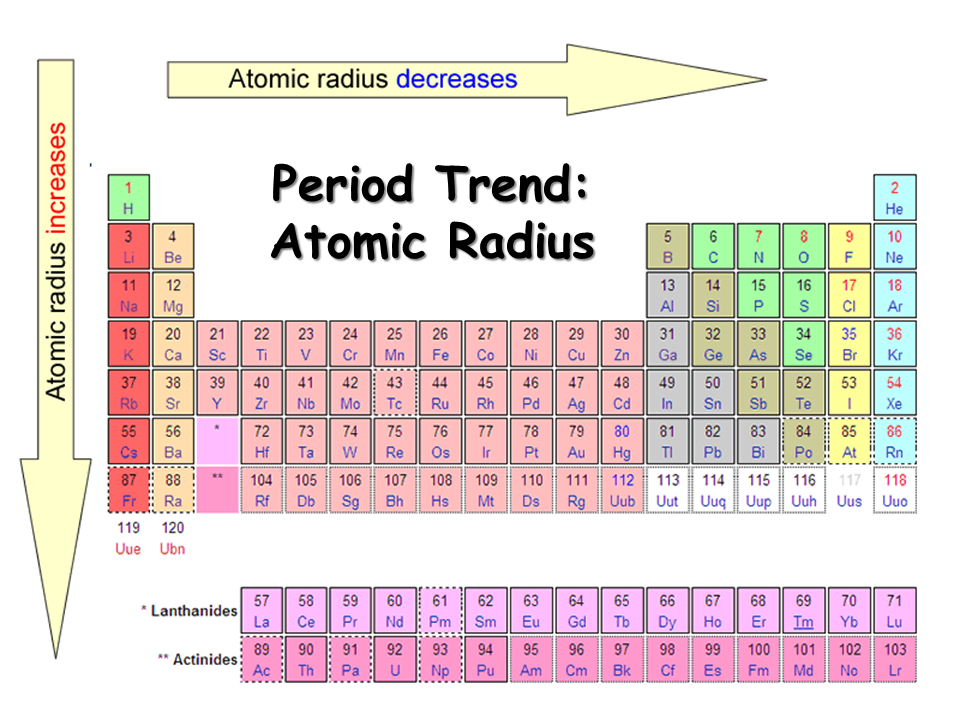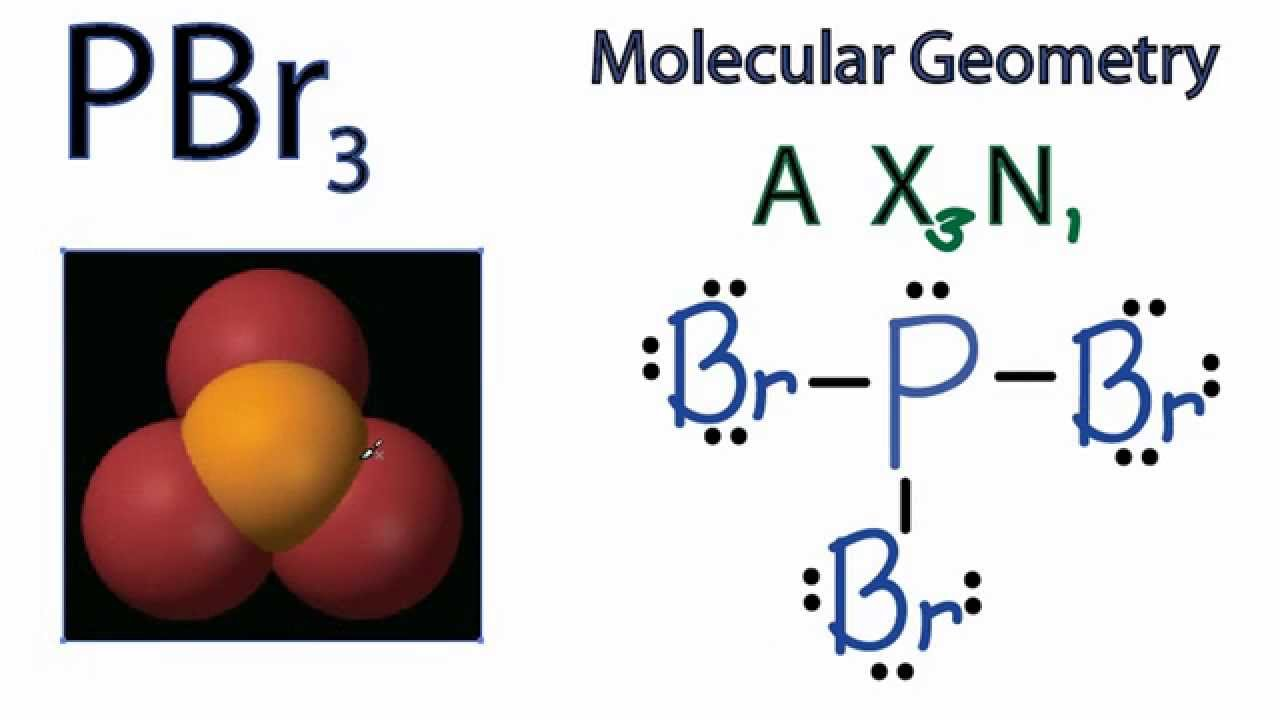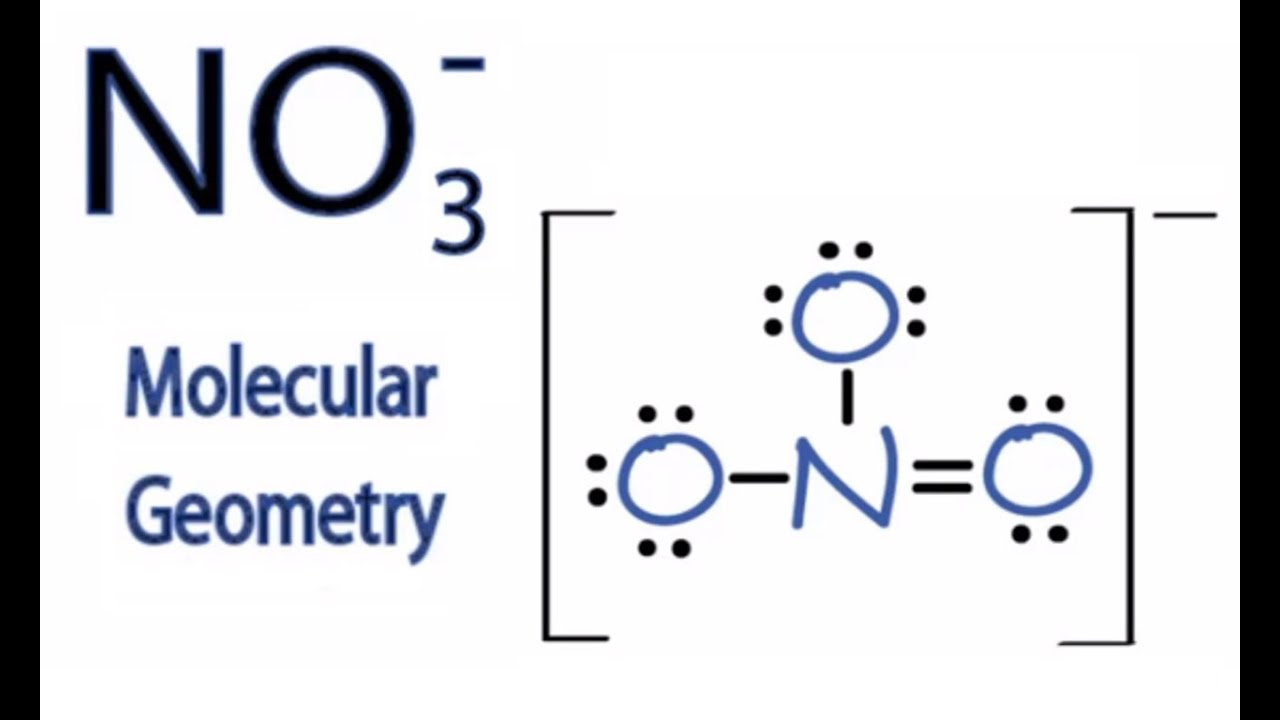Resources:

Have you found videos, websites, or explanations that helped you understand this chapter? Let us know and we'll add them to "Resources" part of this page for other students to use.

Answer Explanations Are Now Available !

Question 1

An ionic substance typically exhibits all of the following properties except

Select one:Explanation

Ionic compounds are polar compounds so they only dissolve in polar solvent.

Question 2

The Lewis structure for Sulfur dioxide can be described by two resonance structures:These resonance structures indicates that the

Select one:Explanation

Resonance structure have bonds that are hybrid cross between a single or double bond.Question 3

Which concept(s) explain why the water molecule is bent and that the angle is about 105º?

Select one:Explanation

Lone pairs have a repulsive force that causes the molecule to bend.

Question 4

Which statement is incorrect about the boiling points for the 6A hydrides: H2O, H2S, H2Se, and H2Te

Select one:Explanation

The smaller the radius the higher the boiling point.

Highest Boiling Point : H2S

Second Highest Boiling Point: H2Se

Third Highest Boiling Point: H2TeQuestion 5

A compound consisting of an element having a low ionization energy and a second element having a large electronegativity is likely to have

Select one:ExplanationQuestion 6

Which of the following molecules is nonpolar?

Select one:ExplanationBased on the Lewis structure if the electrongnegative bond cancels out then it is polar and if it doesn't then it is a polar molecule.

Question 7

The molecule of the type ML4E2 consists of four single bonds and two lone pairs. What structure is it expected to assume?

Select one:ExplanationQuestion 8

The shape that most closely describes the H2S molecule is

Select one:Explanation2 Bonds + 2 lone pairs = Bent

Question 9

In which pair are the molecules geometrically similar?

Select one:Explanation

Shape : 3 bonds  + 2 lone pairs = T-shapedQuestion 10

Which molecule is planar?

Select one:ExplanationQuestion 11

What set of species is arranged in order of increasing S-N-S bond angle?

Select one:Explanation

Remember lone pairs of electrons are bulkier than bonding pairs.

Rule of Thumb: lone pair of electrons have greater repulsive force. The greater the number of lone pairs of electrons the smaller bond angle. The less number of  lone pair electrons the greater the bond angle.

Question 12

What type of hybrid orbital is used in PCl5?

Select one:Explanation5 bonds + 0 lone pair = 5Question 13

The molecule OCNH (shown below) has been detected in gas clouds between stars. The predicted O-C-N bond angle is aboutSelect one:Explanation

O-C-N bond is linear so bond angle is 180 degrees

Question 14

Which element is most likely to form two bonds?

Select one:Explanation

S has 6 valence electrons so it can make 2 bonds

Question 15

How many sigma bonds and pi bonds are in this structure?Select one:Explanation

•  Double Bond = 1 sigma bond + 1 pi bond
• Single Bond = 1 sigma bond

Question 16

Arrange the nitrogen-nitrogen bond lengths in order from shortest to longest for N2, N2H2, N2H4.

Select one:Explanation

N2 : triple bond (shortest)

N2H2: double bond (medium)

N2H: signal bond (longest)

Question 17

What is the formal charge on carbon for the following incorrect Lewis structureSelect one:Explanation

atom's charge = # valence electrons - ( # free electron -  #bonded electrons/2)

C = 4 - 0 - (8/2) = 0

Question 18

What is the formal charge on the right oxygen for the following incorrect Lewis structureSelect one:Explanation

atom's charge = # valence electrons - ( # free electron -  #bonded electrons/2)

O = 6 - 2 - (6/2) =  +1

Question 19Select one:Explanation

isomer : same compound with different arrangement of elements

Question 20

MA2B2 is a molecule where M is the central atom bonded to 2 atoms of A and 2 atoms of B. The fact that it exists in only one form (no isomers) suggests its geometry is

Select one:Explanation

Central atom M: 4 bonds + 0 lone pairs =

Question 21

Which compound would be expected to have the largest dipole moment?
Select one:Explanation3 bonds + 1 lone pair = trigonal pyramidal